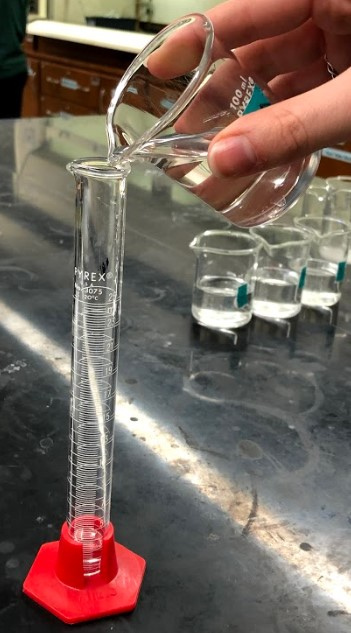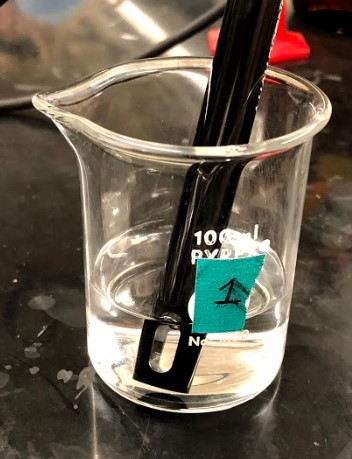# 5: Acid Strength

$$\newcommand{\vecs}{\overset { \rightharpoonup} {\mathbf{#1}} }$$ $$\newcommand{\vecd}{\overset{-\!-\!\rightharpoonup}{\vphantom{a}\smash {#1}}}$$$$\newcommand{\id}{\mathrm{id}}$$ $$\newcommand{\Span}{\mathrm{span}}$$ $$\newcommand{\kernel}{\mathrm{null}\,}$$ $$\newcommand{\range}{\mathrm{range}\,}$$ $$\newcommand{\RealPart}{\mathrm{Re}}$$ $$\newcommand{\ImaginaryPart}{\mathrm{Im}}$$ $$\newcommand{\Argument}{\mathrm{Arg}}$$ $$\newcommand{\norm}{\| #1 \|}$$ $$\newcommand{\inner}{\langle #1, #2 \rangle}$$ $$\newcommand{\Span}{\mathrm{span}}$$ $$\newcommand{\id}{\mathrm{id}}$$ $$\newcommand{\Span}{\mathrm{span}}$$ $$\newcommand{\kernel}{\mathrm{null}\,}$$ $$\newcommand{\range}{\mathrm{range}\,}$$ $$\newcommand{\RealPart}{\mathrm{Re}}$$ $$\newcommand{\ImaginaryPart}{\mathrm{Im}}$$ $$\newcommand{\Argument}{\mathrm{Arg}}$$ $$\newcommand{\norm}{\| #1 \|}$$ $$\newcommand{\inner}{\langle #1, #2 \rangle}$$ $$\newcommand{\Span}{\mathrm{span}}$$$$\newcommand{\AA}{\unicode[.8,0]{x212B}}$$

Objectives

• To determine the difference between a strong and a concentrated acid.
• To determine the difference between a weak and a dilute acid.
• To determine the relationship between ionization and pH.

While pure acids are molecular compounds, when they are placed in water the molecules dissociate and form ionic solutions composed of hydronium ions $$\ce{H_{3}O^{+}}$$ and the counter anion $$\ce{A^{-}}$$.

$\ce{HA} (aq) + \ce{H_{2}O} (l) \ce{<=>} \ce {H_{3}O^{+}} (aq) + \ce {A^{-}} (aq) \label {1}$

The presence of the hydronium ion is the basis for determining the pH of the solution.

$pH=-\log[\ce{H_{3}O^{+}}]\label{2}$

Using the apparatus with the pH sensor attached will allow you to collect data for the value of the pH in each solution. This will give you information on the concentration of hydronium ions in solution.

Conductivity measures the ability of current to be conducted by a solution. Because it is the ions in the solution that carry current, the more ions there are, the higher the conductivity will be. Thus, conductivity is a measure of the number of ions in a solution.

You will be preparing 0.10 M, 0.010 M, 0.0010 M, and 0.00010 M solutions of hydrochloric acid $$\ce{HCl}$$, acetic acid $$\ce{C_{2}H_{4}O_{2}}$$, and mandelic acid $$\ce{C_{8}H_{8}O_{3}}$$.

## Procedure

Safety And Waste Disposal

• The solutions will be sufficiently dilute and as such can be disposed of in the sink.

###### Step 1Label 4 small beakers (100 or 150 mL) 0.10 M (Beaker 1), 0.010M (Beaker 2), 0.0010 M (Beaker 3), and 0.00010 M (Beaker 4). Be sure to identify the formula or name of the acid on each label.

###### Step 2Place 50.0 mL of one of your 0.10 M acid solutions into Beaker 1.

###### Step 3Into each of the three remaining Beakers (2 – 4) add 45 mL of deionized water.

###### Step 4Using a graduated cylinder, add 5.0 mL of the acid in Beaker 1 into Beaker 2 and mix THOROUGHLY.

###### Step 5

Using a graduated cylinder, add 5.0 mL of the acid in Beaker 2 into Beaker 3 and mix THOROUGHLY.

###### Step 6

Using a graduated cylinder, add 5.0 mL of the acid in Beaker 3 into Beaker 4 and mix THOROUGHLY.

Part II:  Measuring pH

Note

• The pH electrode bulbs are made of glass. They are very fragile. Use extreme care.
• Measure the pH of each of your acids three times rinsing the sensor between readings.
###### Step 1Connect the pH Sensor to LabQuest in Channel 1 (CH1).

###### Step 2Use about 100 mL of distilled water in a 250 mL beaker to rinse the electrode.

###### Step 3Starting with the 0.00010 M solution, pour about 10 mL of the test solution into a clean 50 mL beaker and use it to thoroughly rinse the electrode.

###### Step 4Place the pH electrode in the remaining amount of acid in the beaker. Swirl the solution vigorously. Readings may drift without proper swirling! When the pH reading stabilizes, record the value in your notebook.

###### Step 5

Repeat with the remaining solutions to determine pH working from the most dilute to the most concentrated beaker.

###### Step 6When you are finished, rinse the sensor with distilled water and return it to the sensor soaking solution.

Part III:  Measuring Conductivity

Note

You cannot use the conductivity sensor and pH sensor at the same time.

###### Step 1Attach the conductivity sensor to the LabQuest in Channel 2 (CH2). Move the range selector switch on the conductivity sensor box to “0-20000 $$\mu$$S/cm” (the largest range).

###### Step 2Rinse the sensor with distilled water and pat dry. Do not push the paper towel through the cavity in the probe, but gently tap to remove any droplets of water.

###### Step 3Test only the 0.1 M solutions of each acid. Dip the probe into the solution and swirl the solution. Wait 5 seconds for the conductivity to stabilize. Record the value in your notebook.

###### Step 4Rinse and dry the sensor.

## Calculations

###### Step 1

Calculate the average values for the pH of all the acid solutions at each concentration. Record the values on your notebook

###### Step 2

Open Windows Excel and enter the data on initial concentration and pH for the three acids.

###### Step 3

Plot pH vs –log (HA)o for each acid (three plots). Use a linear trend line and add the equation along with the $$R^{2}$$ value, to the graph. Print these plots.

###### Step 4

Calculated the experimental $$[\ce{H_{3}O^{+}}]$$ for all the solutions.

###### Step 5

Calculate % ionization

$\%\: ionization=\frac{[\ce{H_{3}O^{+}}]}{(HA)_{o}}\label{3}$

###### Step 6

Calculate, using the appropriate equations, the theoretical $$[\ce{H_{3}O^{+}}]$$ in each solution. For the weak acids, you will need the values of $$K_{a}$$ you found in prelab.

###### Step 7

Calculate the theoretical pH based on the theoretical $$[\ce{H_{3}O^{+}}]$$

5: Acid Strength is shared under a not declared license and was authored, remixed, and/or curated by LibreTexts.